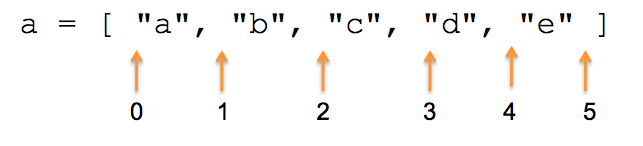## Arrays

Ref. WGR Chapter 9, Section 9.2, Collection handling with arrays

## Array

• sized dynamically
• can contain mixed types
• zero-indexed
• can be defined literally (inline) e.g.
``````fruits = ["apple", "banana"]
``````

## Arrays act like stacks

``````a = [1, 2, 3]
a.push "four" #=> [1, 2, 3, "four"]
a.pop         #=> "four"
a             #=> [1, 2, 3]
``````

## or like queues

``````a = [1, 2, 3]
a.push(4)        #=> [1, 2, 3, 4]
a.shift          #=> 1
a                #=> [1, 2, 3]

a.unshift "zero" #=> ["zero", 1, 2, 3]
``````
• `push` means "enqueue"; `shift` means "dequeue"

## or like sets

``````a = [1, 2, 3]
a.include?(2)  #=> true
``````

(technically an array is not a set because it doesn't enforce uniqueness)

## `first` and `last`

``````a          #=> 1
a.first       #=> 1
a[a.size-1]   #=> 3
a.last        #=> 3
``````

## Adding to an array

``````a = []
a << "x"       #=> ["x"]
``````

`<<` (pronounced "shovel") does a `push` -- adds an item to the end of an array -- DESTRUCTIVE!

``````a = []
a + ["x"]       #=> ["x"]
``````

`+` does a `concat` -- adds two arrays together and makes a new array -- safe!

## plus-equals

• for Strings and Arrays, plus-equals uses `concat`, not `append`
• that means it makes a copy, but then reassigns the variable
• so it feels destructive, but isn't
``````a = ["x", "y"]

a + "z"        #=> can't convert String into Array
a + ["z"]      #=> ["x", "y", "z"] (but a is unchanged)
a += ["z"]     #=> ["x", "y", "z"] (and a is changed)

a = b = ["x"]
a += ["y"]
a #=> ["x", "y"]
b #=> ["x"]
``````

## `size` isn't everything

• `size` and `length` are synonyms
• both give you the number of items in the array

## Accessing array items

Arrays are zero-indexed

``````fruit = ["apple", "banana", "cherry"]
fruit #=> "apple"
fruit #=> "cherry"
fruit #=> nil
``````

## Zero Is Better Than One

• If you use 0-based indexing

• and you always use less than, not <=
• then you never have to add or subtract one
• so you have less change of an off-by-one error
• Think of the index as pointing to the space between items• This allows consistent length and looping semantics
• It's always "less than the limit"
• and "the limit is the size"
• i.e. a[0,3] has 3 things in it, indexed 0,1,2

## Fun with Array Indexes

``````fruit = ["apple", "banana", "cherry", "date"]
``````

negative indexes count from the back

``````fruit[-1] #=> "date"
fruit[-3] #=> "banana"
``````

range indexes

``````fruit[1..3]  #=> ["banana", "cherry", "date"]
fruit[1...3] #=> ["banana", "cherry"]
``````

## getting out of bounds

``````>> a = ["apple", "banana", "cherry"]
=> ["apple", "banana", "cherry"]
>> a.length
=> 3
>> a
=> nil
>> a.size
=> 3
>> a
=> ["apple", "banana", "cherry"]
``````

moral: getting past the end returns `nil`, not error

## setting out of bounds

``````>> a
=> ["apple", "banana", "cherry"]
>> a = "jicama"
=> "jicama"
>> a.size
=> 10
>> a
=> ["apple", "banana", "cherry", nil, nil,
nil, nil, nil, nil, "jicama"]
``````

moral: setting past the end autofills with `nil`

## Multidimensional arrays (aka matrices)

• No special matrix syntax
• Have to be built up "by hand" as arrays of arrays
``````times_table = []
4.times do |x|
times_table[x] = []
4.times do |y|
times_table[x][y] = x * y
end
end

>> times_table
=> [[0, 0, 0, 0], [0, 1, 2, 3], [0, 2, 4, 6], [0, 3, 6, 9]]
>> times_table
=> 6
>> times_table
=> [0, 2, 4, 6]

``````